# Chapter 2 – Introduction to Numbering Systems

## 2.1  Decimal (base-10)

The numbering system we use is called the decimal system.  It consists of 10 numbers: 0123456789.  Some ancient civilizations used other numbering systems.  For example, the Babylonians used the sexagesimal system, based on the number 60.  The Babylonians divided the circle into 360 degrees (a multiple of 60).  The Mayas used the vigesimal system, based on the number 20.

## 2.2  Unary (base-1)

A base-1 numbering system was used by some people in ancient times before more advanced systems were developed.  In a base-1 system, you simply put a mark (or a 1) for each item you are counting.  For example, bones have been found with 29 and 30 notches, possibly corresponding to the cycle of the moon: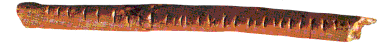Placing a mark for every item you are counting would be quite cumbersome if you need to count larger quantities.  Slightly more advanced are numbering systems that have special symbols to represent larger numbers.  For example, Roman numerals use I, V, X, etc.  The ancient Egyptians also used symbols to represent larger quantities.  The number below is 2,382,358.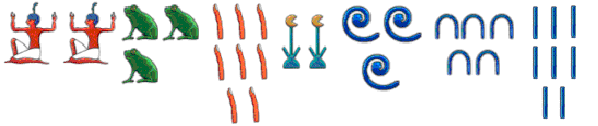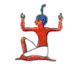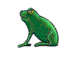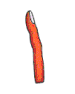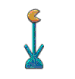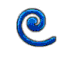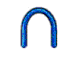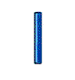1,000,000 100,000 10,000 1,000 100 10 1

## 2.3  Binary (base-2)

The electronic circuitry within computers is composed of millions of transistors.  Each of these circuits has two states – on and off.  On and off is viewed as representing 1 and 0, respectively.  Because of this, a base-2 numbering system was devised for computers.  Counting in binary is often a tricky concept to understand for newcomers.

In binary, each digit has only two numbers – 0 and 1.  With a two-digit decimal number, you can count from 0 to 99.  With a two-digit binary number, you can only count to 3.  The following table shows all the combinations of two binary digits with their decimal equivalent.

 2-Digit Binary number Decimal Equivalent 00 0 01 1 10 2 11 3

One method that might help you understand counting in binary would be to use the decimal system, but only keep the numbers that contain 0's and 1's.  The following table shows these numbers in bold:

 0 1 2 3 4 5 6 7 8 9 10 11 12 13 14 15 16 17 18 19 20 21 22 23 24 25 26 27 28 29 30 31 32 33 34 35 36 37 38 39 40 41 42 43 44 45 46 47 48 49 50 51 52 53 54 55 56 57 58 59 60 61 62 63 64 65 66 67 68 69 70 71 72 73 74 75 76 77 78 79 80 81 82 83 84 85 86 87 88 89 90 91 92 93 94 95 96 97 98 99 100 101 102 103 104 105 106 107 108 109 110 111 112 113 114 115 116 117 118 119

With a four-digit binary number, you can count from 0 to 15.  The following table shows all the combinations of four binary digits with their decimal equivalent.

 Binary Decimal Binary Decimal 0000 0 1000 8 0001 1 1001 9 0010 2 1010 10 0011 3 1011 11 0100 4 1100 12 0101 5 1101 13 0110 6 1110 14 0111 7 1111 15

There are different methods used to convert between binary and decimal numbers.  One way to convert a binary number to decimal is to calculate the value of each digit and add these up.  Since binary is based on multiples of 2, each successive digit is two times the previous.  The following example illustrates this.

Value of each digit:       8  4  2  1                       Add 2 + 8

↓  ↓  ↓  ↓

Binary number:             1  0  1  0                       Decimal number: 10

In a binary number, the first digit (farthest to the right) has a value of 1.  The next digit (to the left) has a value of 2.  The third digit has a value of 4.  The fourth digit has a value of 8, etc.  In the binary number 1010, since the second and fourth digits are 1, you would add 2 + 8 which is equal to 10.  Look at another example.

Value of each digit:       16  8  4  2  1                 Add 1 + 2 + 4 + 16

↓   ↓  ↓  ↓  ↓

Binary number:              1   0  1  1  1                 Decimal number: 23

A bit is a single binary digit, which can be 0 or 1.  A byte is 8 binary digits (8 bits), which allows you to count from 0 to 255.  Data in most computers is organized in bytes.  The following table shows how the metric system applies to bits and bytes.

 Unit Size What it Can Store bit 0 or 1 One bit of information can store answers to yes/no questions: "Do you have a driver’s license?" byte 8 bits Each character in a document on your computer uses one byte of storage in ASCII, or two bytes in Unicode. kilobyte (KB) 1,000 bytes 2 kilobytes could store one page of a document.  50 Kilobytes can store a low resolution photograph. megabyte (MB) 1,000,000 (million) bytes 1 megabyte can store a novel.  5 Megabytes can store the complete works of Shakespeare or 30 seconds of TV-quality video.  640 megabytes can store a music CD. gigabyte (GB) 1,000,000,000 (billion) bytes 1 gigabyte can store a movie at TV quality.  4 gigabytes can store a DVD movie. terabyte (TB) 1,000,000,000,000 (trillion) bytes 10 terabytes can store the printed section of the Library of Congress. petabyte (PB) 1015 bytes 350 petabytes is the size of all photos uploaded to Facebook as of 2013. exabyte (EB) 1018 bytes 10 exabytes is an estimate of the entire size of Google's datacenters in 2013. zettabyte (ZB) 1021 bytes In 2013, the entire World Wide Web is estimated at 4 zettabytes. yottabyte (YB) 1024 bytes

Hexadecimal was developed as an easier way to deal with binary.  With four binary digits, you can count from 0 to 15 in decimal (as shown in the table in the previous section).  One hexadecimal digit can be used to replace four binary digits.  Hexadecimal uses the numbers 0 – 9, then A – F to give a total of 16 numbers.

 Decimal 0 1 2 3 4 5 6 7 8 9 10 11 12 13 14 15 Binary 0000 0001 0010 0011 0100 0101 0110 0111 1000 1001 1010 1011 1100 1101 1110 1111 Hexadecimal 0 1 2 3 4 5 6 7 8 9 A B C D E F

As can be seen in the previous table, the 16 different hexadecimal digits are 0123456789ABCDEF.  When counting in hex, you combine the numbers similar as to counting in decimal.  The next numbers after F are 10, 11, 12, 13, 14, 15, 16, 17, 18, 19, 1A, 1B, 1C, 1D, 1E, 1F, 20, 21, etc.  Often, a hexadecimal number is written with a 0x in front of it: 0xB means B hex, or 11 in decimal.

## 2.5  ASCII

In most computers, each character of data is stored using one byte (8 bits) of memory.  Each space and punctuation mark also uses one byte.  Therefore, this sentence takes 40 bytes.  Since each byte represents a number between 0 and 255, ASCII is way of coding data so that each character has a corresponding number.  For example, "a" is 97;  "b" is 98, "c" is 99, etc.  The following ASCII chart shows each character and the corresponding number used to represent it.

 ASCII Chart ASCII# Char ASCII# Char ASCII# Char ASCII# Char ASCII# Char ASCII# Char 0 – 8 unused 53 5 78 N 103 g 128 Ç 153 Ö 9 tab 54 6 79 O 104 h 129 ü 154 Ü 10 line feed 55 7 80 P 105 i 130 é 155 ¢ 11 - 31 unused 56 8 81 Q 106 j 131 â 156 £ 32 space 57 9 82 R 107 k 132 ä 157 ¥ 33 ! 58 : 83 S 108 l 133 à 158 ₧ 34 " 59 ; 84 T 109 m 134 å 159 ƒ 35 # 60 < 85 U 110 n 135 ç 160 á 36 \$ 61 = 86 V 111 o 136 ê 161 í 37 % 62 > 87 W 112 p 137 ë 162 ó 38 & 63 ? 88 X 113 q 138 è 163 ú 39 ' 64 @ 89 Y 114 r 139 ï 164 ñ 40 ( 65 A 90 Z 115 s 140 î 165 Ñ 41 ) 66 B 91 [ 116 t 141 ì 166 ª 42 * 67 C 92 \ 117 u 142 Ä 167 º 43 + 68 D 93 ] 118 v 143 Å 168 ¿ 44 , 69 E 94 ^ 119 w 144 É 169 ⌐ 45 - 70 F 95 _ 120 x 145 æ 170 ¬ 46 . 71 G 96 ` 121 y 146 Æ 171 ½ 47 / 72 H 97 a 122 z 147 ô 172 ¼ 48 0 73 I 98 b 123 { 148 ö 173 ¡ 49 1 74 J 99 c 124 | 149 ò 174 « 50 2 75 K 100 d 125 } 150 û 175 » 51 3 76 L 101 e 126 ~ 151 ù 52 4 77 M 102 f 127 ⌂ 152 ÿ

You will notice in this ASCII chart that there are many characters (e.g. Æ ) that do not have a key on the keyboard.  These characters can be typed by holding down the Alt key and typing the ASCII number on the keypad.  For example, open up the Windows notepad program.  Hold the Alt key and type "171" on the keypad.  When you release the Alt key, the character "½" will appear.

## 2.6  Storing Text on a Computer

The table below illustrates how the letter ‘Z’ is stored on a computer electronically.

 The letter ‘Z’ is typed on the computer using a word processor. Z The letter ‘Z’ is converted to a number using the ASCII table.  Decimal 90 corresponds to the letter ‘Z’. 90 The decimal number 90 is converted to binary (01011010). 01011010 In the computer’s memory, there are electronic circuits that are either on or off.  One circuit is used to represent one binary digit – 1 if the circuit is on, 0 if the circuit is off.  Eight of these circuits form one byte.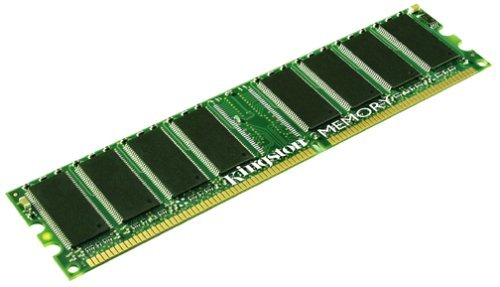Off-On-Off-On-On-Off-On-Off

## Exercises – Chapter 2

1.   Convert the following binary numbers to decimal:

(a) 11   (b) 0101  (c) 1111   (d) 10101   (e) 11111111   (f) 11111110

2.   Convert the following decimal numbers to binary:

(a) 5   (b) 2   (c) 7   (d) 8   (e) 15   (f) 24

3.   Convert the following hexadecimal numbers to binary:

(a) 1   (b) 9   (c) B   (d) DC   (e) F1   (f) FF

4.   List the ASCII numbers for the following words:

(a) Hi       ___ ___

(b) apple    ___ ___ ___ ___ ___

(c) kiwi     ___ ___ ___ ___

(d) Excite!  ___ ___ ___ ___ ___ ___ ___

5.   What do the following ASCII Numbers translate to?

73 32 97 109 32 104 117 110 103 114 121 46

6.   If a photograph is 700KB in size, how many could you store on a
2 gigabyte flash drive?